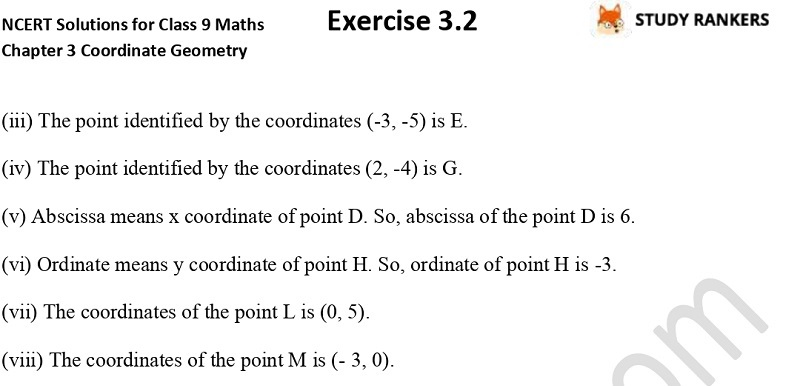>

## NCERT Solutions for Class 9 Maths Chapter 3 Coordinate Geometry Exercise 3.2

Want to access NCERT solutions of Chapter 3 Coordinate Geometry Exercise 3.2 then you can easily get them here. Class 9 Maths NCERT Solutions can help you in ascertaining answers of difficult questions easily so you can complete your homework easily. These NCERT answers are updated as per the latest marking scheme released by CBSE.

Exercise 3.2 has only two questions in which you have to name horizontal and the vertical lines drawn to determine the position of any point in the Cartesian plane, What is the name of each part of the plane formed by these two lines etc.X